# Predicate Logic or FOL Chapter 8 Propositional Logic

• Slides: 34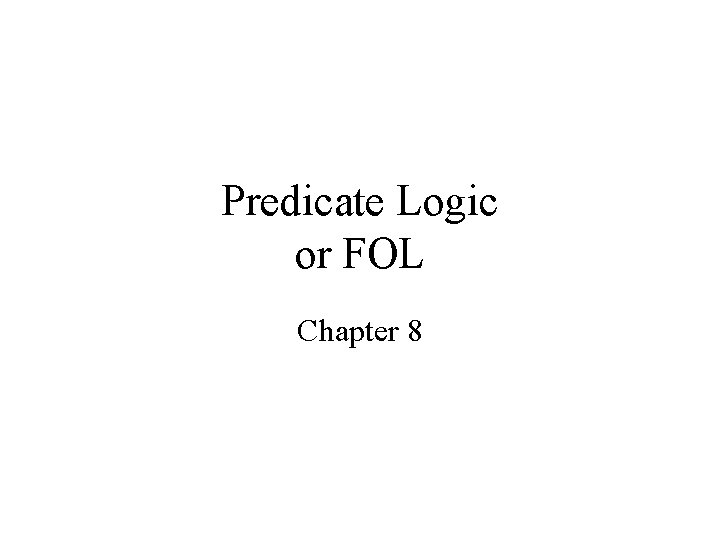Predicate Logic or FOL Chapter 8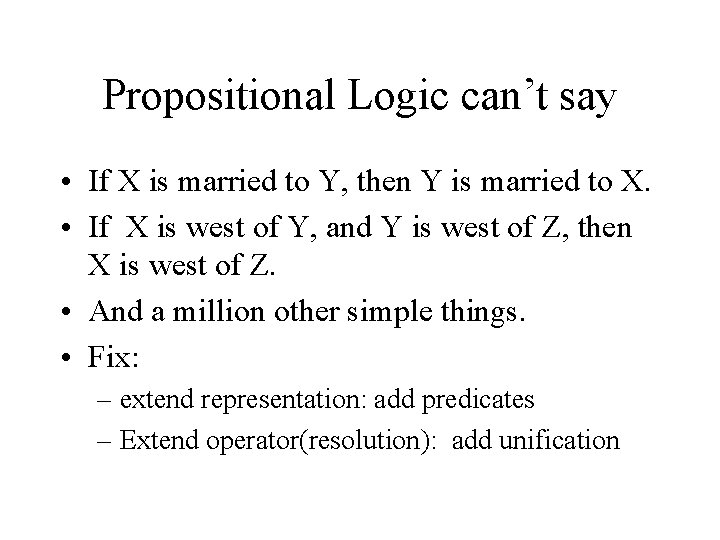Propositional Logic can’t say • If X is married to Y, then Y is married to X. • If X is west of Y, and Y is west of Z, then X is west of Z. • And a million other simple things. • Fix: – extend representation: add predicates – Extend operator(resolution): add unification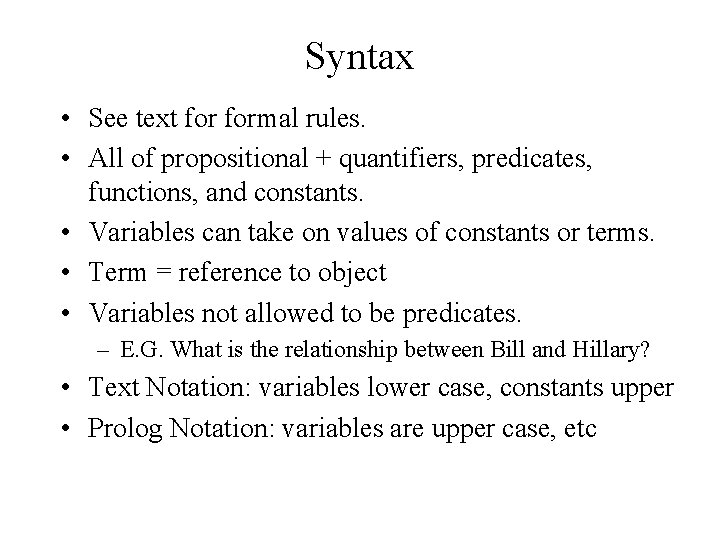Syntax • See text formal rules. • All of propositional + quantifiers, predicates, functions, and constants. • Variables can take on values of constants or terms. • Term = reference to object • Variables not allowed to be predicates. – E. G. What is the relationship between Bill and Hillary? • Text Notation: variables lower case, constants upper • Prolog Notation: variables are upper case, etc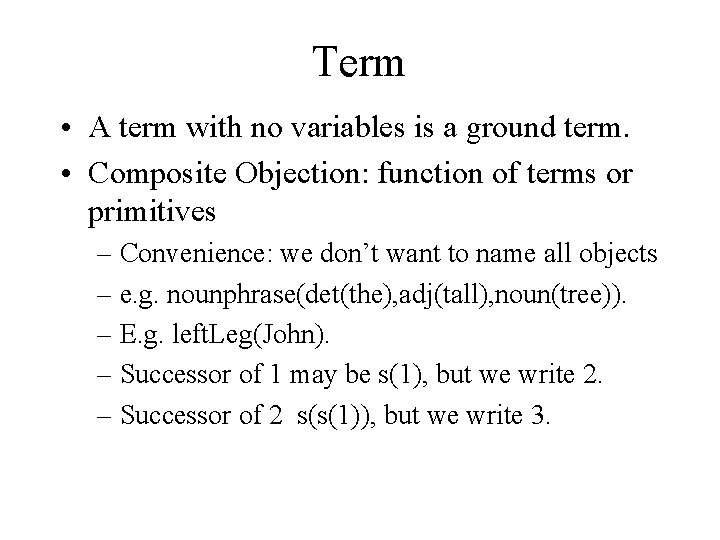Term • A term with no variables is a ground term. • Composite Objection: function of terms or primitives – Convenience: we don’t want to name all objects – e. g. nounphrase(det(the), adj(tall), noun(tree)). – E. g. left. Leg(John). – Successor of 1 may be s(1), but we write 2. – Successor of 2 s(s(1)), but we write 3.Goldbach’s Conjecture • For all n, if integer(n), even(n), greater(n, 2) then there exists p 1, p 2, integer(p 1), integer(p 2), prime(p 1), prime(p 2), and equals(n, sum(p 1, p 2)). • Quantifiers: for all, there exists • Predicates: integer, greater, prime, even, equals. • Constants: 2 • Functions: sum.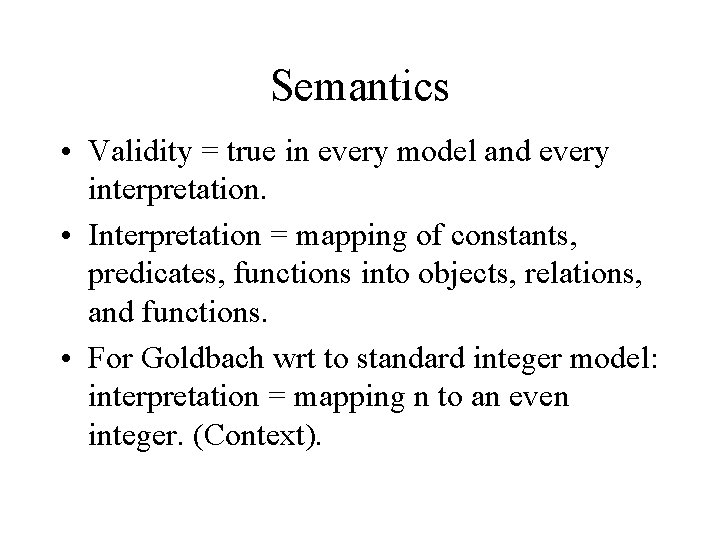Semantics • Validity = true in every model and every interpretation. • Interpretation = mapping of constants, predicates, functions into objects, relations, and functions. • For Goldbach wrt to standard integer model: interpretation = mapping n to an even integer. (Context).Representing World in FOL • All kings are persons. goes to? • for all x, King(x) & Person(x). • for all x, King(x) => Person(x).Representing World in FOL • All kings are persons. • for all x, King(x) => Person(x). OK. • for all x, King(x) & Person(x). Not OK. – this says every object is a king and a person. • In Prolog: person(X) : - king(X). • Everyone Likes icecream. • for all x, Likes(x, icecream).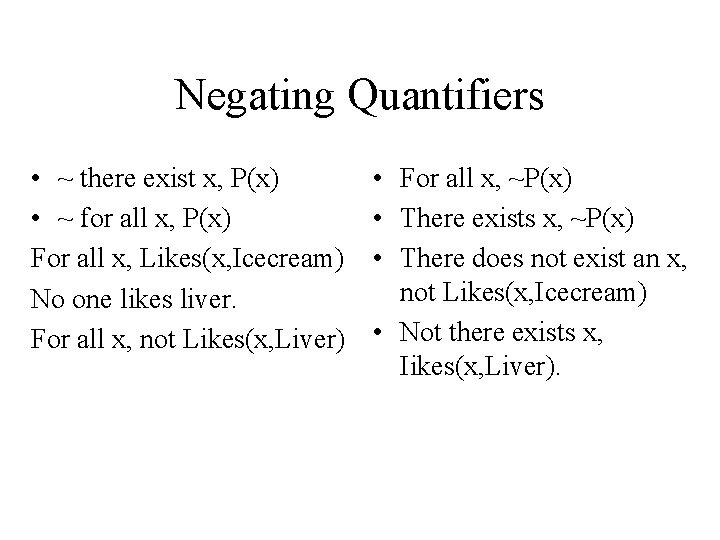Negating Quantifiers • ~ there exist x, P(x) • ~ for all x, P(x) For all x, Likes(x, Icecream) No one likes liver. For all x, not Likes(x, Liver) • For all x, ~P(x) • There exists x, ~P(x) • There does not exist an x, not Likes(x, Icecream) • Not there exists x, Iikes(x, Liver).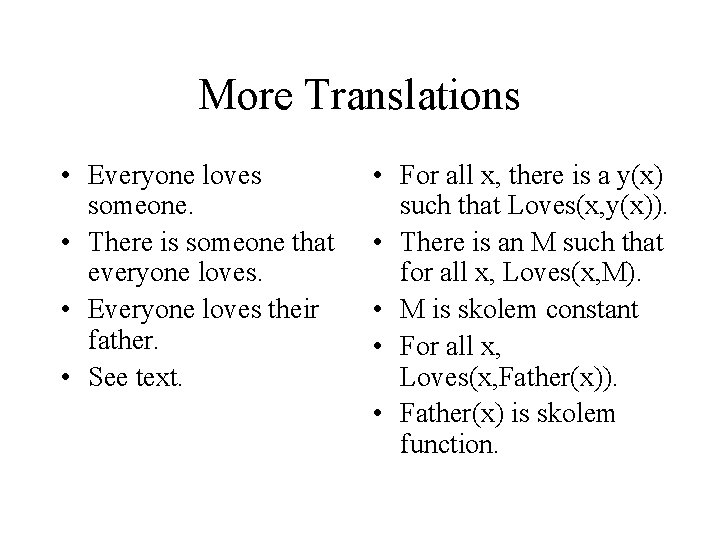More Translations • Everyone loves someone. • There is someone that everyone loves. • Everyone loves their father. • See text. • For all x, there is a y(x) such that Loves(x, y(x)). • There is an M such that for all x, Loves(x, M). • M is skolem constant • For all x, Loves(x, Father(x)). • Father(x) is skolem function.Unification • If p and q are logical expressions, then Unify(p, q) = Substitution List S if using S makes p and q identical or fail. • Standardize apart: before unifying, make sure that p and q contain different variable names.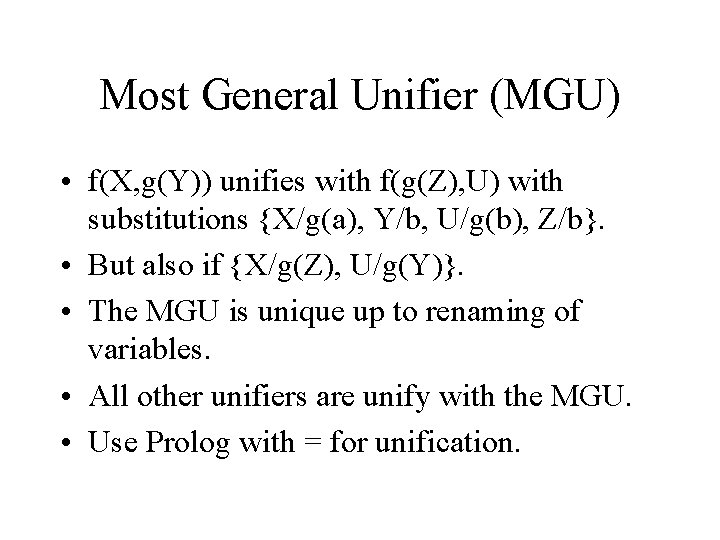Most General Unifier (MGU) • f(X, g(Y)) unifies with f(g(Z), U) with substitutions {X/g(a), Y/b, U/g(b), Z/b}. • But also if {X/g(Z), U/g(Y)}. • The MGU is unique up to renaming of variables. • All other unifiers are unify with the MGU. • Use Prolog with = for unification.Occurs Checking • When unifying a variable against a complex term, the complex term should not contain the same variable, else non-match. • Prolog doesn’t check this. • Ex. f(X, X) and f(Y, g(Y)) should not unify.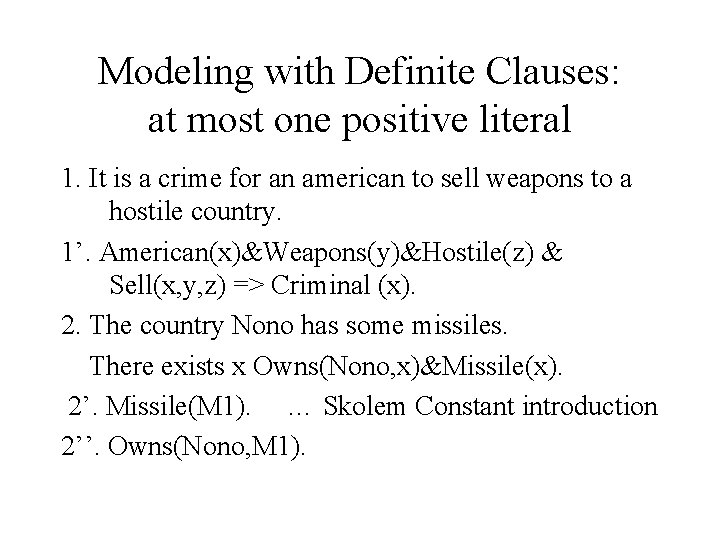Modeling with Definite Clauses: at most one positive literal 1. It is a crime for an american to sell weapons to a hostile country. 1’. American(x)&Weapons(y)&Hostile(z) & Sell(x, y, z) => Criminal (x). 2. The country Nono has some missiles. There exists x Owns(Nono, x)&Missile(x). 2’. Missile(M 1). … Skolem Constant introduction 2’’. Owns(Nono, M 1).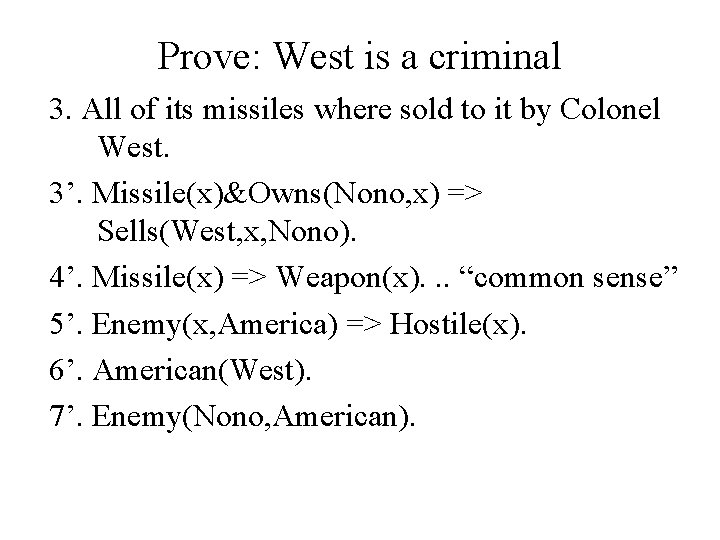Prove: West is a criminal 3. All of its missiles where sold to it by Colonel West. 3’. Missile(x)&Owns(Nono, x) => Sells(West, x, Nono). 4’. Missile(x) => Weapon(x). . . “common sense” 5’. Enemy(x, America) => Hostile(x). 6’. American(West). 7’. Enemy(Nono, American).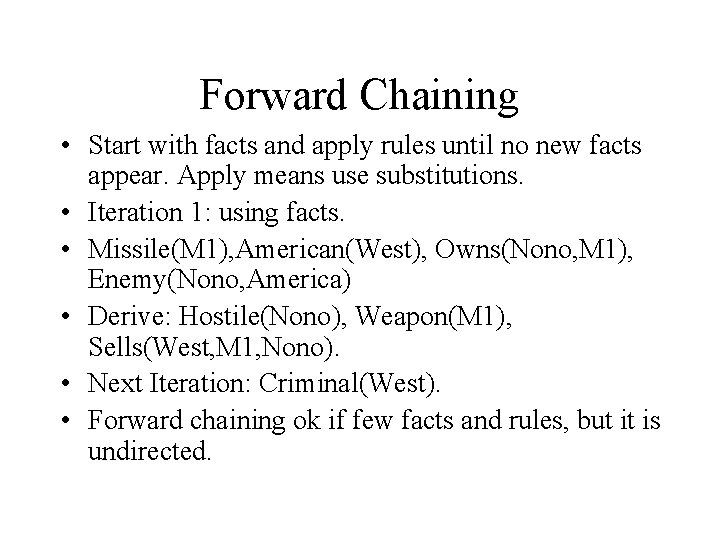Forward Chaining • Start with facts and apply rules until no new facts appear. Apply means use substitutions. • Iteration 1: using facts. • Missile(M 1), American(West), Owns(Nono, M 1), Enemy(Nono, America) • Derive: Hostile(Nono), Weapon(M 1), Sells(West, M 1, Nono). • Next Iteration: Criminal(West). • Forward chaining ok if few facts and rules, but it is undirected.Resolution gives forward chaining • Enemy(x, America) =>Hostile(x) • Enemy(Nono, America) • |- Hostile(Nono) • Not Enemy(x, America) or Hostile(x) • Enemy(Nono, America) • Resolve by {x/Nono} • To Hostile(Nono)Backward Chaining • Start with goal, Criminal(West) and set up subgoals. This ends when all subgoals are validated. • Iteration 1: subgoals American(x), Weapons(y) and Hostile(z). • Etc. Eventually all subgoals unify with facts.Resolution yeilds Backward Chaining • A(x) &W(y)&H(z)& S(x, y, z) =>C(x) • -A(x) or –W(y) or –H(z) or –S(x, y, z) or C(x). • Add goal –C(West). • Yields –A(West) or -W(y) or –H(z) or -S(West, y, z). Etc.Resolution is non-directional • Both a power (inference representation) and a weakness (no guidance in search) • -a or –b or –c or d or e equals • a, b, c =>d or e and • a, b, c, -d => e etc. • Prolog forces directionality and results in an incomplete theorem prover.FOL -> Conjuctive Normal Form • • • Similar to process for propositional logic, but Use negations rules for quantifiers Standarize variables apart Universal quantification is implicit. Skolemization: introduction of constants and functions to remove existential quantifiers.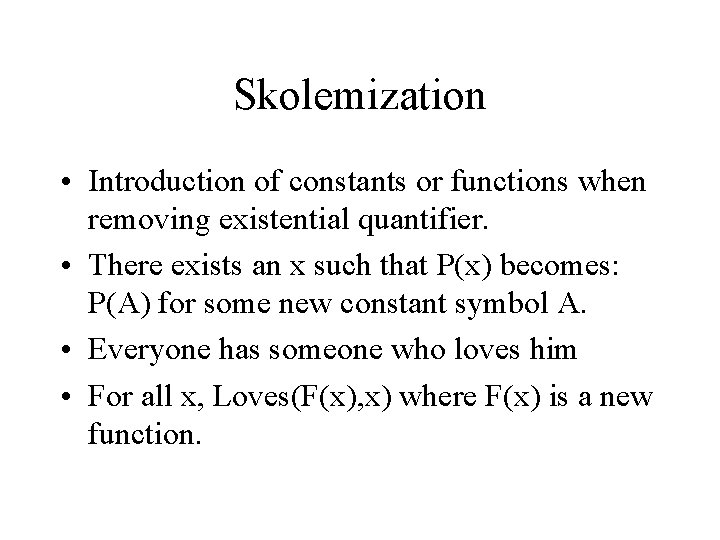Skolemization • Introduction of constants or functions when removing existential quantifier. • There exists an x such that P(x) becomes: P(A) for some new constant symbol A. • Everyone has someone who loves him • For all x, Loves(F(x), x) where F(x) is a new function.Resolution in CNF • Just like propositional case, but now complimentary first order literals unify. • Theorem (skipping proof): FOL with resolution is refutation complete, i. e. if S is a set of unsatisfiable clauses, then a contradiction arises after a finite number of resolutions. • Let’s take in on faith!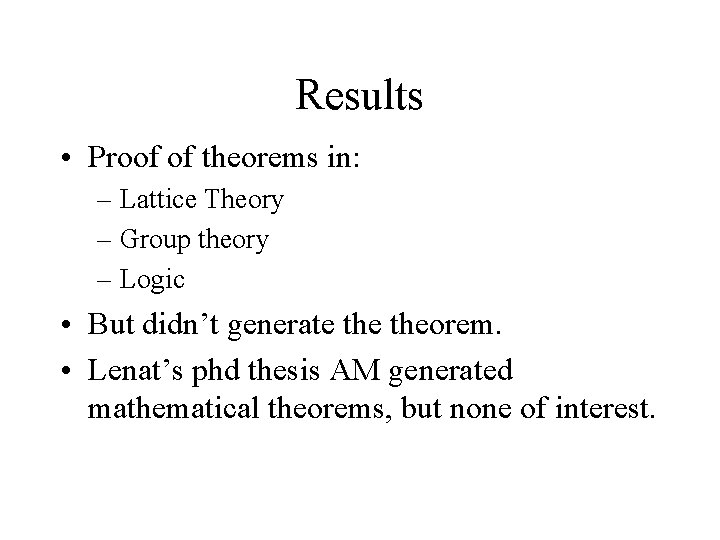Results • Proof of theorems in: – Lattice Theory – Group theory – Logic • But didn’t generate theorem. • Lenat’s phd thesis AM generated mathematical theorems, but none of interest.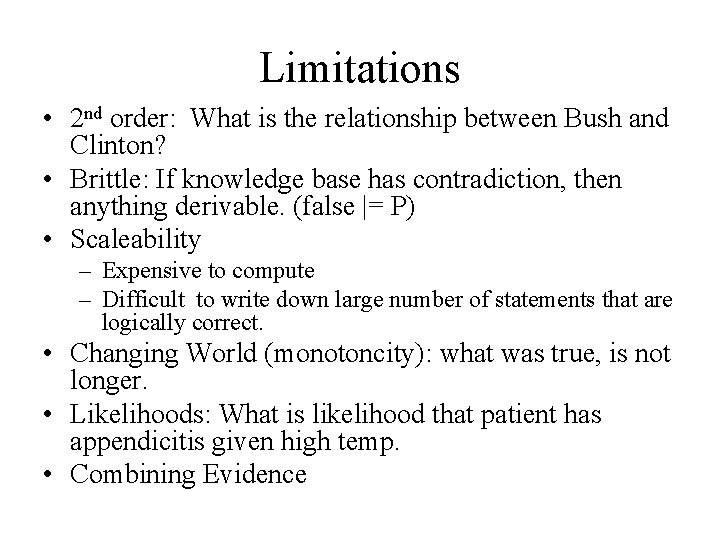Limitations • 2 nd order: What is the relationship between Bush and Clinton? • Brittle: If knowledge base has contradiction, then anything derivable. (false |= P) • Scaleability – Expensive to compute – Difficult to write down large number of statements that are logically correct. • Changing World (monotoncity): what was true, is not longer. • Likelihoods: What is likelihood that patient has appendicitis given high temp. • Combining Evidence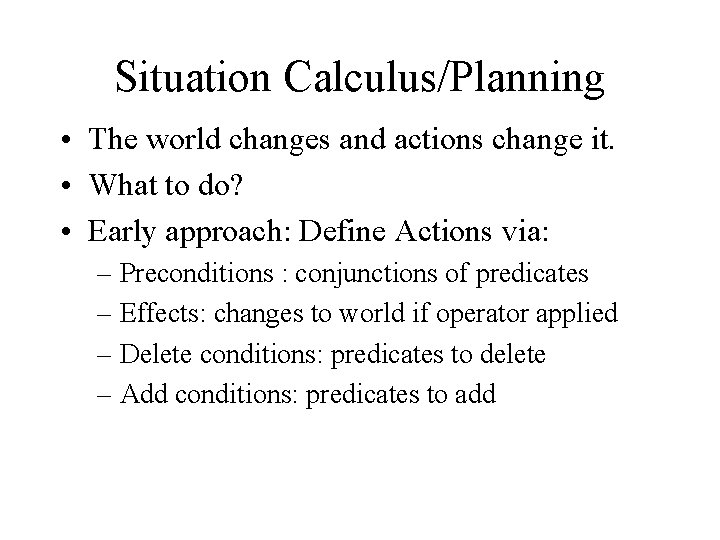Situation Calculus/Planning • The world changes and actions change it. • What to do? • Early approach: Define Actions via: – Preconditions : conjunctions of predicates – Effects: changes to world if operator applied – Delete conditions: predicates to delete – Add conditions: predicates to addBlocks World Example • Action: Move(b, x, y)…move b from x to y • Preconditions: – On(b, x)&Clear(y)&Block(b)& Clear(b) – Careful: and b = y else problems ( b to b) • Postconditions: – On(b, y) & Clear(x) & not On(b, x) & not Clear(y) • Similar for other operators/actions. • Now search: better plan searchers possible.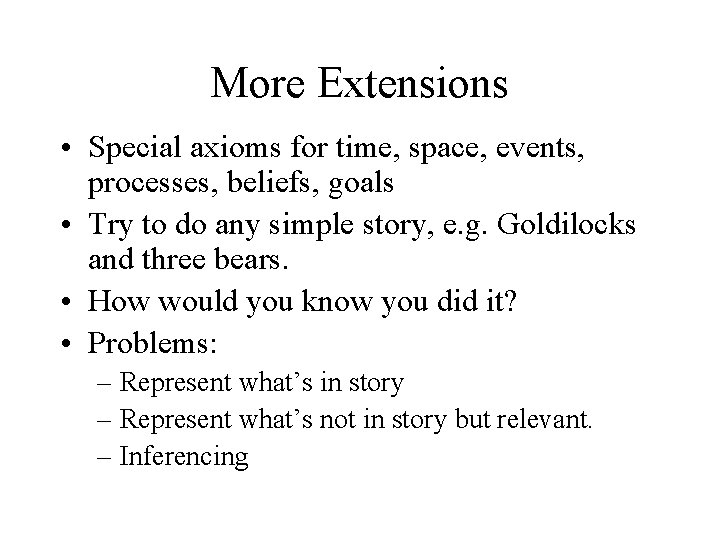More Extensions • Special axioms for time, space, events, processes, beliefs, goals • Try to do any simple story, e. g. Goldilocks and three bears. • How would you know you did it? • Problems: – Represent what’s in story – Represent what’s not in story but relevant. – InferencingTime • Before (x, y) implies After(y, x) • After(x, y) imples Before(y, x) • Before(x, y) and Before(y, z) implies Before(x, z) etc. • When are you done? • What about during?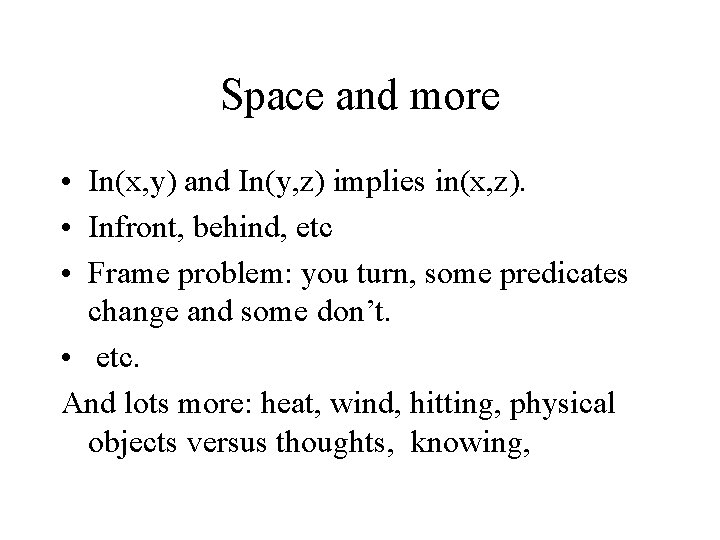Space and more • In(x, y) and In(y, z) implies in(x, z). • Infront, behind, etc • Frame problem: you turn, some predicates change and some don’t. • etc. And lots more: heat, wind, hitting, physical objects versus thoughts, knowing,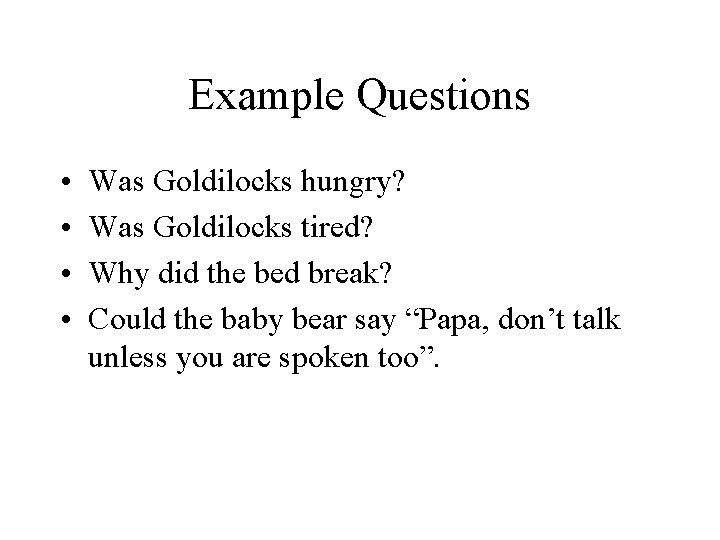Example Questions • • Was Goldilocks hungry? Was Goldilocks tired? Why did the bed break? Could the baby bear say “Papa, don’t talk unless you are spoken too”.Expert Systems: Engineering Approach • We can keep the representation language of FOL, but do not adopt the semantics. • Attach to each fact and rule a belief (#) • Provide an ad hoc calculus for combining beliefs. • Now multiple proofs valuable since they will add evidence. • This worked, if domain picked carefully. The hard part: getting the rules or knowledge.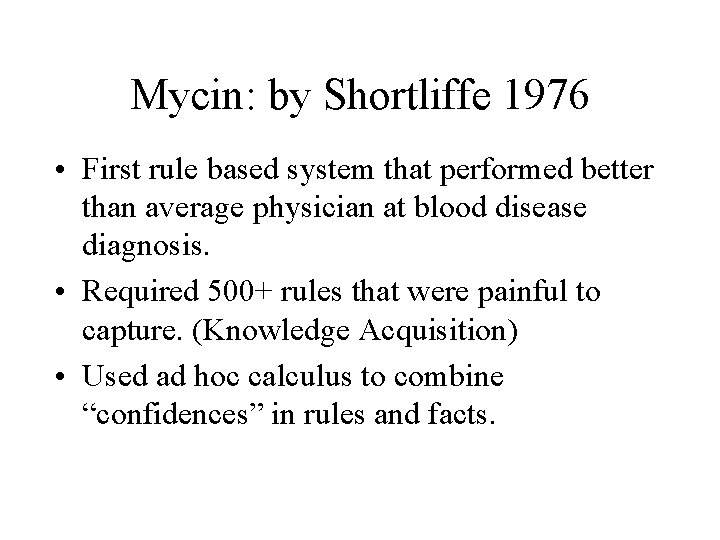Mycin: by Shortliffe 1976 • First rule based system that performed better than average physician at blood disease diagnosis. • Required 500+ rules that were painful to capture. (Knowledge Acquisition) • Used ad hoc calculus to combine “confidences” in rules and facts.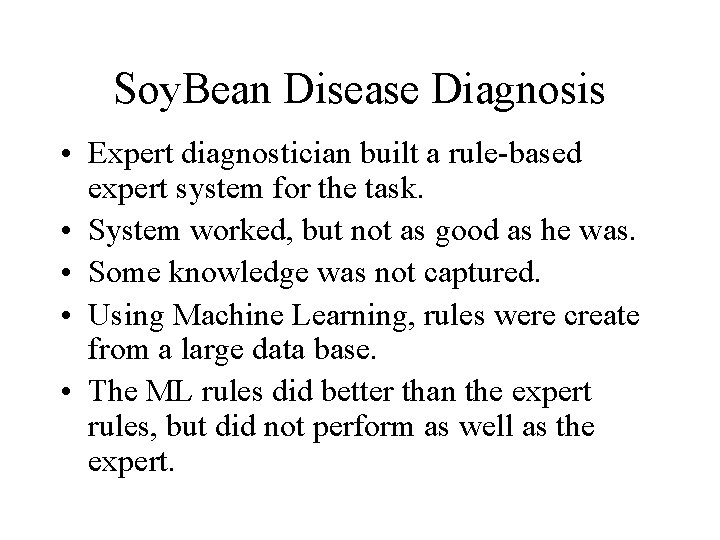Soy. Bean Disease Diagnosis • Expert diagnostician built a rule-based expert system for the task. • System worked, but not as good as he was. • Some knowledge was not captured. • Using Machine Learning, rules were create from a large data base. • The ML rules did better than the expert rules, but did not perform as well as the expert.